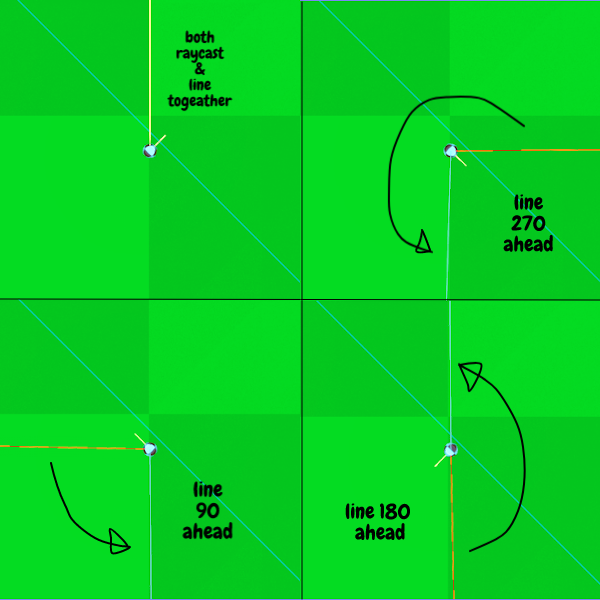issue Solved!

I'm trying to draw a line with ImmediateGeometry from the origin of the im node(or the player node or raycast node, there all in the same position) to the collision point of the raycast, the line draws fine, but when the player is rotated the line seems to rotate twice as much as the player does, so for a 360 rotation of the player the line will have rotated 720just ignore that small line coming out from the ball thats the balls raycast.

heres the code im using to draw the line

``````func get_preview_path():
var raycast = self
var im = \$ImmediateGeometry
var normal
var start = im.transform.origin
var collision_point
if raycast.is_colliding():
im.clear()
normal = get_collision_normal()
collision_point = get_collision_point()

im.begin(1,null)
im.set_color(Color(1,1,1,1))
im.set_normal(Vector3(0,-1,0))

im.set_color(Color(1,1,1,1))
im.set_normal(Vector3(0,-1,0))
im.end()
``````

the getpreviewpath func is called from the player.gd in the player rotation func

``````func player_rotate():

var rotate_strength = 0.1
var left = Input.is_action_pressed("player_rot_left")
var right = Input.is_action_pressed("player_rot_right")
if left:
self.rotation_degrees.y += rotate_strength
Global.Ball.rotation_degrees.y += rotate_strength
\$RayCast.get_preview_path()
elif right:
self.rotation_degrees.y -= rotate_strength
Global.Ball.rotation_degrees.y -= rotate_strength
\$RayCast.get_preview_path()
``````

im pretty sure PI radians plays a part here, but i have no idea...
if anyone could point me in the right direction, i would be very much appreciative.

in Engine
edited

+1 vote

If you're rotating the player, any child of the player will also rotate. This means that if you're getting the player's rotation and using it to draw an angled line, that line will also rotate. ie. Player is rotated 45deg, line is drawn at a 45deg angle, then gets rotated 45deg, which causes the line to appear to be rotated 90deg.

In this case, you have 3 options:

1. Move the ImmediateGeometry so that it is no longer a child of your player node.
2. When drawing the line, do it along the axis your player faces when not rotated.
3. Rotate the ImmediateGeometry in the opposite direction the same amount that the player is rotated.
by (56 points)

Thank you!
moving ImmediateGeometry out from player and into the level scene fix the problem
i will try the other 2 solutions later, but for now it works as i expected it to work.
Thanks again!